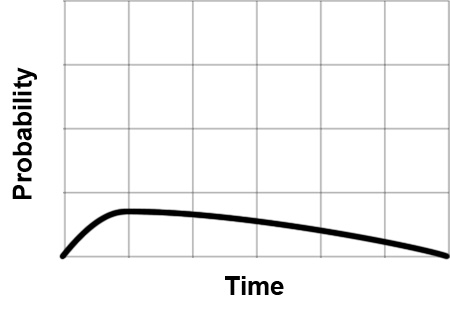# Working with probability over time

I am looking for some help with the math for firing an event at some point over a period of time.

Assume I want a light to change from green to red, at some point between 2 and 30 seconds. I need to make it much more likely to happen at 5 seconds than at 25 seconds, but also much more likely to happen at 5 seconds than at 2 seconds. Ideally the increases are logarithmic rather than linear. The chance of the light changing is 100%; when it happens is all that is being determined.I will be doing this work with JavaScript.

I hope I worded this well, and thank you in advance for any insight.

You can simulate the curve with a few line segments, using Lerp to interpolate the values in each segment. Let’s suppose you want 3 segments, from 2 to 4, 4 to 6 and 6 to 30:

``````function ChangeColorAfterDelay(){
var x = Random.Range(0.0, 3.0); // get a float value between 0 and 3
var t: float;
if (x < 1.0){ // first segment
t = Mathf.Lerp(2, 4, x);
} else if (x < 2.0){ // second segment
t = Mathf.Lerp(4, 6, x-1.0);
} else { // third segment
t = Mathf.Lerp(6, 30, x-2.0);
}
Invoke("ChangeColor", t); // change the color after the random delay t
}

function ChangeColor(){
light.color = Color.red;
}
``````

This code gives equal chances to the 3 segments: 33% for [2-4[, 33% for [4-6[, 33% for [6-30]. The basic idea may be used for more than 3 segments, and each segment may be redefined to get different distributions.

So I’d say the best way would be to define a variable on your script like this:

``````  var curve : AnimationCurve;
``````

Setup the curve like this perhaps, using the Inspector:

[5038-screen+shot+2012-11-18+at+22.22.46.png|5038]

The find out the amount of time like this:

``````    var timeToChange = curve.Evaluate(Random.value * curve[curve.length-1].time);
``````

The curve here hangs around 5 seconds for most of the range. You can make the curve as wide as you like (the random is multiplied out to take account of that).

A specular lighting trick to get fall-off curves from 0-1 is `pow(random(0,1), P);`. Random 0-1 squared starts at 0, stays near the bottom, and curves up to 1. The larger the value of P, the more it “likes” to hang around at 0. But the range is always the full 0-1 (0.999 to the 7th is still pretty much 1.)

To turn that into a 5-30 range, `T = 5+25*pow(random.value, 4)`

To get the fall-off going back to 2, maybe `T=5-3*pow(random.value, 9);` and flip a coin for which one to use.